# 4.5 Contact Self-Energies

Boundary conditions have to be specified to model the contacts, which act as a source or drain for electrons. While the matrix representing the HAMILTONian of the device has a finite dimension, the total HAMILTONian matrix is infinite dimensional due to the semi-infinite contacts.

The influence of the contacts can be folded into the device region. Due to the transitions between the device and the contacts, the influence of the contacts can be demonstrated by adding contact self-energies to the total self-energy . The self-energy matrices for the contacts and the HAMILTONian matrix for the device have the same rank, but the self-energy matrices are highly sparse. For example, only one carbon ring at the source end of the channel couples to the source, thus only one sub-matrix is non-zero for the source self-energy. Similarly, only one sub-matrix is non-zero for the drain self-energy. As shown in Appendix G, non-zero blocks of the contact self-energies are given by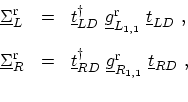(4.27)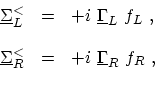(4.28)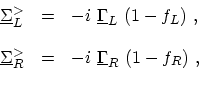(4.29)

where sub-scripts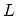and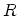denote the left (source) and right (drain) contacts, respectively,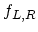are the FERMI factor of the contacts,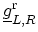are the surface GREEN's function of the contacts, and finally the broadening functions are defined as(4.30)

Surface GREEN's functions can be calculated using a recursive relation described in Appendix G.3. In this section two types of contacts are discussed: semi-infinite CNTs acting as the source and drain contacts and SCHOTTKY type metal-CNT contacts. The respective surface GREEN's functions and self-energies for the both contact types are derived next.

In mode-space representation (see Section 4.4) the matrices in (4.27) to (4.30) become one-dimensional. Thus, the respective quantities for each mode can be treated as numbers and the computational cost decreases considerably.
Subsections

M. Pourfath: Numerical Study of Quantum Transport in Carbon Nanotube-Based Transistors